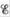# Consider the following galvanic cell at 25°C: Pt | Cr 2+ (0 .30 M ),Cr 3+ (2 .0 M ) | | Co 2+ (0 .20 M ) | Co The overall reaction and equilibrium constant value are 2 Cr 2 + ( a q ) + Co 2 + ( a q ) → 2 Cr 3 + ( a q ) + Co ( s ) K = 2.79 × 10 7 Calculate the cell potential, for this galvanic cell and Δ G for the cell reaction at these conditions.### Chemistry: An Atoms First Approach

2nd Edition
Steven S. Zumdahl + 1 other
Publisher: Cengage Learning
ISBN: 9781305079243

#### Solutions

Chapter
Section### Chemistry: An Atoms First Approach

2nd Edition
Steven S. Zumdahl + 1 other
Publisher: Cengage Learning
ISBN: 9781305079243
Chapter 17, Problem 87E
Textbook Problem
563 views

## Consider the following galvanic cell at 25°C: Pt | Cr 2+ (0 .30 M ),Cr 3+ (2 .0 M ) | | Co 2+ (0 .20 M ) | Co The overall reaction and equilibrium constant value are 2 Cr 2 + ( a q ) + Co 2 + ( a q ) → 2 Cr 3 + ( a q ) + Co ( s )         K =   2.79   ×   10 7 Calculate the cell potential,for this galvanic cell and ΔG for the cell reaction at these conditions.

Interpretation Introduction

Interpretation:

A reaction taking place in a galvanic cell and the equilibrium constant for the same reaction is given. The value of cell potential E and ΔG is to be calculated.

Concept introduction:

The relationship between reduction potential and standard reduction potential value and activities of species present in an electrochemical cell at a given temperature is given by the Nernst equation.

The value of Ecell is calculated using Nernst formula,

E=E°(RTnF)ln(Q)

At room temperature the above equation is specifies as,

E=E°(0.0591n)log(Q)

This relation is further used to determine the relation between ΔG° and K , ΔG° and E°cell .

To determine: The value of cell potential E and ΔG for the given cell reaction.

### Explanation of Solution

Given

The cell reaction is,

Pt|Cr2+(0.30M),Cr3+(2.0M)||Co2+(0.20M)|Co

The value of K is 2.79×107 .

The reaction taking place at cathode is,

Co2+(aq)+2eCo(s)E°red=0.28V

The reaction taking place at anode is,

Cr2+(aq)Cr3+(aq)+eE°ox=0.41V

Multiply oxidation half reaction with the coefficient of 2 and add both the reduction and oxidation half-reaction,

Co2+(aq)+2eCo(s)2Cr2+(aq)2Cr3++2e

The final equation is,

2Cr2+(aq)+Co2+(aq)2Cr3+(aq)+Co(s)

The overall cell potential is calculated as,

E°cell=E°ox+E°red=0.41V+(0.28V)=0.13V

The reaction involves the transfer of 2 moles of electrons.

The cell potential is calculated using the Nernst equation,

E=E°(0.0591n)log(Q)

Where,

• E is the cell potential.
• E° is the cell potential at the standard conditions.
• Q is the activity of the species in the cell.
• n is the number of electrons involved in the reaction.

Substitute the values of Eo , n and Q in above equation as,

E=0

### Still sussing out bartleby?

Check out a sample textbook solution.

See a sample solution

#### The Solution to Your Study Problems

Bartleby provides explanations to thousands of textbook problems written by our experts, many with advanced degrees!

Get Started

Find more solutions based on key concepts
A deficiency of niacin may result in which disease? pellagra beriberi scurvy rickets

Nutrition: Concepts and Controversies - Standalone book (MindTap Course List)

Is the light chain of an immunoglobulin the same as the V region?

Introduction to General, Organic and Biochemistry

Identify and describe the tissues shown below.

Human Biology (MindTap Course List)

A young woman buys an inexpensive used car stock car racing. It can attain highway speed with an acceleration o...

Physics for Scientists and Engineers, Technology Update (No access codes included)